﻿ Polynomial Simulation and Refutation of Complex Formulas of Resolution over Linear Equations in Propositional Proof System

### Polynomial Simulation and Refutation of Complex Formulas of Resolution over Linear Equations in Prop...

Vishwa Nath Maurya, Avadhesh Kumar MauryaOPEN ACCESSPEER-REVIEWED

## Polynomial Simulation and Refutation of Complex Formulas of Resolution over Linear Equations in Propositional Proof System

Vishwa Nath Maurya1,, Avadhesh Kumar Maurya2

1Professor & Principal, Shekhawati Engineering College, Rajasthan Technical University, India

2Assistant Professor, Department of Electronics & Communication Engineering Lucknow Institute of Technology, U.P. Technical University, Lucknow, India

### Abstract

In this paper, we present that the propositional proof system R(lin) (Resolution over Linear Equations) established by Ran Raz and Iddo Tzameret is not a super system, there exists a sequence of tautologies, which require proof complexity exponential in size of tautologies. We show that there are the sequence of unsatisfiable collections of disjuncts of linear equations, which require exponential lower bounds in R(lin) and have polynomially bounded refutations by incorporating renaming inference rule to R(lin) system. Some additional properties of R(lin) have been described that many of the “hard” provable in R outstanding examples of propositional tautologies (contradictions) have polynomially bounded proofs in R(lin).

• Maurya, Vishwa Nath, and Avadhesh Kumar Maurya. "Polynomial Simulation and Refutation of Complex Formulas of Resolution over Linear Equations in Propositional Proof System." American Journal of Modeling and Optimization 2.1 (2014): 34-38.
• Maurya, V. N. , & Maurya, A. K. (2014). Polynomial Simulation and Refutation of Complex Formulas of Resolution over Linear Equations in Propositional Proof System. American Journal of Modeling and Optimization, 2(1), 34-38.
• Maurya, Vishwa Nath, and Avadhesh Kumar Maurya. "Polynomial Simulation and Refutation of Complex Formulas of Resolution over Linear Equations in Propositional Proof System." American Journal of Modeling and Optimization 2, no. 1 (2014): 34-38.

 Import into BibTeX Import into EndNote Import into RefMan Import into RefWorks

### 1. Introduction

The research work by some noteworthy researchers found in the literature of propositional calculus shows that there is an increasing demand to innovate and explore the propositional proof system in connection with resolution over linear equations-R(lin). The classical propositional calculus has an underserved reputation among logicians as being essentially trivial, but very common problem of propositional proof complexity presents some of the most intriguing problems in modern logic. In this direction, the starting points of propositional proof complexity was examined by Cook and Reckhow , wherein propositional proof systems have been dignified as polynomial-time computable functions, which have as their range the set of all propositional tautologies. Moreover, Cook and Reckhow  detected also a fundamental connection between lengths of proofs and the separation of complexity classes: they explored that there exists a propositional proof system, which has polynomial-size proofs for all tautologies (a polynomially bounded proof system, which is called super system), if the class NP is closed under complementation. From this observation the so called Cook-Reckhow programme was derived, which suffices as one of the foremost inspirations for propositional proof complexity: to separate NP from coNP (and hence P from NP) it suits to show super-polynomial lower bounds to the size of proofs in all propositional proof systems.

Several noteworthy researchers (e.g. [1-10] ) and references therein focused their attention on the subject. Since in the late of sixth decade, Tseitin  pronounced the first super-polynomial lower bound to the lengths of proofs for resolution; therefore the resolution system is not a super system yet resolution system is one of the most frequently used systems for automated theorem proving. Although, the resolution system is popular in propositional calculus mainly because of its key striking feature of single inference rule and due to popularity, the resolution method is generally used and it can overcome its incompetence in providing proofs of counting arguments. Now there are many proof systems which are generalizations of resolution system such as Res(k) (Resolution with bounded conjunction) presented in Krajicek , SR (Resolution with substitution) introduced in Chubaryan et al. , R(lin) (Resolution over Linear Equations) introduced in Raz and Tzameret  etc. It is well-known that some of tautologies can be presented in different forms: varieties of disjunctive normal form (DNF), conjunctive normal form (CNF), systems of linear inequalities, collections of disjuncts of linear equations etc.

In the present paper, our main objective is to explore some additional properties of R(lin) that many of the “hard” demonstrable formulas in R; outstanding examples of propositional tautologies (contradictions) have polynomially bounded proofs in R(lin). We show that there are the sequence of tautologies consisting of two presentations of negation of which one as the systems of disjuncts of linear equations, based on CNF and the other also as the unsatisfiable collections of disjuncts of linear equations are “hard” refutable in R(lin). In addition to this, we introduce the proof system R(lin)+renaming and examine that the second contra-dictionary collections have polynomially bounded refutations in it. Note that the proof systems considered in this paper intend to prove the unsatisfiability over 0,1 values of collections of disjunctions of linear equations. In other words, proofs in such proof systems intend to refute the collections of clauses, which is to validate their negation, therefore we shall sometimes speak about refutations and proofs interchangeably.

### 2. Preliminary Ideas and Notations

In this paper, the current concept of the unit Boolean cube (En), a propositional formula, a tautology and a proof system have been used as key mathematical tools for Classical Propositional Logic (CPL) and proof complexity.

By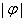we denote the size of a formula φ (or some its presentation), defined as the number of all variable entries. It is obvious that the full length of a formula, which is understood to be the number of all symbols or the number of all entries of logical signs, is bounded by some linear function in.

A tautology φ is called minimal iff φ is not an instance of a shorter tautology.

Moreover, we use the following proof systems;

2.1. Resolution Refutation System

Let us describe the resolution refutation system (R) following . A clause is a disjunction of literals (variables or negated variables). A conjunctive normal form (CNF) formula is a conjunction of clauses.

Resolution is complete and sound proof system for unsatisfiable CNF formulas. Let C and D be two clauses containing neither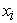nor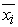. The resolution rule allows one to derive C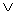D from C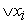and D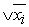.

The weakening rule allows deriving the clause CD from the clause C for any two clauses C, D.

2.1.1. Definition 1 (Resolution)

A resolution proof of the clause D from a CNF formula K is a sequence of clauses D1, D2, … , Dl such that:

i. Each clause Dj is either a clause of K or can be obtained from two previous clauses in the sequence using the resolution rule or weakening rule.

ii. The last clause Dl = D.

A resolution refutation of a CNF formula K is a resolution proof of the empty clause from K (the empty clause stands for FALSE, that is no value satisfies to the empty clause).

2.2. Resolution over Linear Equations

Let us describe R(lin) system following Pudlak . R(lin) is an extension of well-known resolution, which operates with disjunction of linear equations with integer coefficients. A disjunction of linear equations is of the following form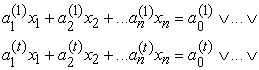where t ≥ 0 and the coefficients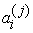are integers (for all 0 ≤ i n 1 ≤ j t). We discard duplicate linear equations from a disjunction of linear equations. Any CNF formula can be translated into a collection of disjunctions of linear equations directly: every clause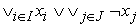(where I and J are sets of indices of variables) involved in the CNF is translated into the disjunction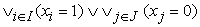. For a clause D we denote by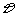its translation into a disjunction of linear equations. It is fairly easy to verify that any Boolean assignment of the variables x1, …, xn satisfies a clause D iff it satisfies.

As we wish to deal with Boolean values, we augment the system with axioms, called Boolean axioms: (xi = 0) ∨ (xi = 1) for all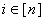.

Axioms are not fixed: for any formula φ we obtain ¬φ, then we obtain R(lin) translation of CNF of ¬φ. We also add Boolean axioms for each variable of φ.

2.2.1. Definition 2 (R(lin))

Let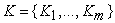be a collection of disjunctions of linear equations. An R(lin)- proof from K of a disjunction of linear equations D is a finite sequence D1, …, Dl of disjunctions of linear equations such that Dl = D and for every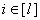, either Di = Kj for some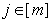, or Di is a Boolean axiom (xh = 0) ∨ (xh = 1) for some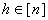, or Di was deduced by one of the following R(lin)-inference rules, using Dj, Dk for some j, k < i.

Resolution: Let A, B be two disjunctions of linear equations (possibly the empty disjunctions) and let L1, L2 be two linear equations. From A L1 and B L2 it is derived A B ∨ (L1 + L2) ( +resolution) or A B ∨ (L1 - L2) (-resolution).

Weakening: From a disjunction of linear equations A derive A L, where L is an arbitrary linear equation.

Simplification: From A ∨ (0 = k) derive A, where A is a disjunction of linear equations and (k ≠ 0).

An R(lin) refutation of a collection of disjunctions of linear equations K is a proof of the empty disjunction from K. Raz and Tzameret showed that R(lin) is a sound and complete Cook-Reckhow refutation system for unsatisfiable CNF formulas (translated into unsatisfiable collection of disjunctions of linear equations).

Really, if we use the “- resolution” rule and “simplification” rule (instead of resolution rule) to two disjunctions of linear equations, which are above described translations from clauses of literals C and D , then we obtain the R(lin)-proof.

2.3. Polynomial Simulation

In the theory of proof complexity two main characteristics of the proof are: t complexity, defined as the number of proof steps, and ℓ– complexity, defined as total number of proof symbols. Let Φ be a proof system and φ be a tautology. We denote by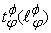the minimal possible value of t – complexity (ℓ – complexity) for all proofs of tautology φ in Φ..

Let Φ1 and Φ2 be two different proof systems. FolloFollowing Cook and Reckhow  we recall

2.3.1. Definition 3

Φ2 p-t-simulates (p- ℓ -simulates) Φ1, if there exists a polynomial p() such that for each formula φ, derivable both in Φ1 and Φ2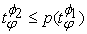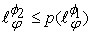.

2.3.2. Definition 4

The systems Φ1 and Φ2 are p-t-equivalent (p- ℓ -equivalent) iff Φ1 p-t-simulates (p- ℓ - simulates) Φ2 and Φ2 p-t-simulates (p- -simulates) Φ1.

2.3.3. Definition 5

The system Φ2 has exponential ℓ - speed-up (t-speed-up) over the system Φ1, if Φ2 p- ℓ - simulates (p-t-simulates) Φ1, and there exists a sequence of such formulas φn, that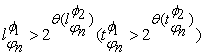It is known that PHPn (the Pigeonhole Principal Tautologies), Tsmodp(n) (Tseitin modp Tautologies), Cliquen,k (the Clique-coloring Principle Tautologies) require exponential t – complexities and ℓ – complexities in R, for more details, we refer Pudlak .

Basing on presentation of mentioned formulas as some collections of disjuncts of linear equations and using in addition the “+ resolution” rule, Raz and Tzameret  confined their attention to explore that they have polynomially bounded proof-complexities in R(lin).

### 3. Sample of Complex Tautologies

In this next section, we investigate the sequence of tautologies, CNF of negations for every of which, translated into unsatisfiable collection of disjuncts of linear equations, as well as some other presentations of these contradictions also as collection of disjuncts of linear equations, require exponential proof-complexity in R(lin). This fact points on some weakness of R(lin). In this direction, Aleksanyan and Chubaryan  paid their attention to contribute the following notes;

We call a replacement-rule each of the following trivial identities for a propositional formula ψ: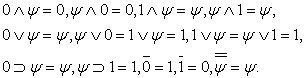Application of a replacement-rule to some word consists in the replacing of some its sub words, having the form of the left-hand side of one of the above identities, by the corresponding right-hand side.

Let φ be a propositional formula,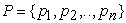be the set of all variables of φ, and let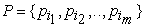(1 ≤ j ≤ m) be some subset of P.

3.1. Definition 6

Given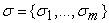Em, the conjunct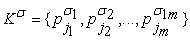is called φ-1- determinative (φ-0-determinative) if assigning σj (1 ≤ j ≤ m) to each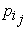and successively using replacement-rule, we obtain the value of φ (1 or 0) independently of the values of the remaining variables.

In further consideration the following tautologies (Topsy- Turvy Matrix) play key role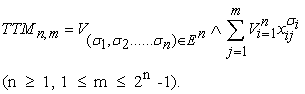For all fixed n ≥ 1 and m in above-indicated intervals every formula of this kind expresses the following true statement: given a 0,1-matrix of order n x m we can “topsy-turvy” some strings (writing 0 instead of 1 and 1 instead of 0) so that each column will contain at least one 1.

3.2. Definition 7

We call the minimal possible number of variables in a φ-determinative conjunct the determinative size of φ and denote it by d(φ).

Obviously, d(φ) < for every formula φ, and the smaller is the difference between these quantities, the “harder” can be considered the formula under study.

3.3. Definition 8

Let φn (n ≥ 1) be a sequence of minimal tautologies. If for some n0 there is a constant c such that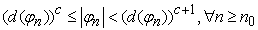then the formulas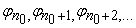are called hard-determinable.

Let φn = TT for all n ≥ 1. Taking into consideration that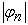= n(2n-1) 2n and d(φn) = 2n, it is not difficult to see, that φ3, φ4, … are hard-determinable.

Note that the formulas PHPn and “Cliquen,k” are not hard-determinable for all values of n since d(PHPn) = 2 and d(“Cliquen least,k”) = 3.It is not difficult to see that the formulas Tsmodp(n) are also not hard-determinable. In  it is proved that CNF of ¬TTMn,m has at least 2m disjuncts, every of which contains m literals, therefore we have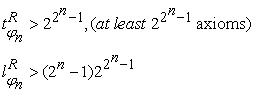If we take above described translation of CNF of ¬φn into collections of disjuncts of linear equations, then the number of axioms, which must be used in R(lin) refutation is at least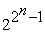, therefore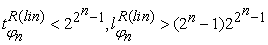But we can consider the other presentation for CNF of ¬φn also as unsatisfiable collections of disjuncts of linear equations.

So, ¬TTMn,m expresses the following contra-dictionary statement:

There exists a 0, 1 – matrix of order n x m (n 1, 1 m 2n-1) such that by every “topsy-turvy” some strings, at least one column consists only of 0.

Or the equivalent statement:

There exists a 0, 1 – matrix of order n x m (n 1, 1 m 2n-1) such that by every “topsy-turvy” some strings, at least for one column the sum of elements is 0.

The statement can be presented by formula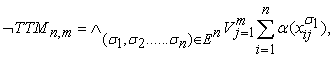Where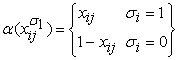This presentation is the collection of disjuncts of linear equations already. After several arithmetical transformations we have simpler equations.

Let us consider the collection of linear equations for ¬TTM’2,3.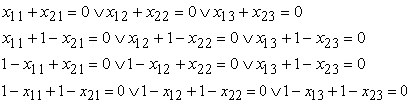or alternatively one can get following system (1)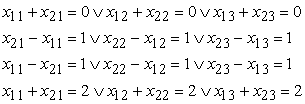As R(lin) axioms for refutation of collection (1) we must take each of linear equations from collection (1) and for every variable xij(i = 1,2; j = 1,2,3) the axiom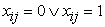In order to refute collection (1) we must obtain from mentioned axioms the equation 0 = k for some integer k, therefore after some steps of refutation we must obtain shorter unsatisfiable equations. It is not difficult to see that every application of inference rule to mentioned axioms gives either satisfiable equation, or longer equation, hence in order to refute collection (1) we must use the following statement (Lemma 4 from )

Let K be a collection of disjunctions of linear equations, and let z abbreviate some linear form with integer coefficients. Let E1, …, E be ℓ disjunctions of linear equations. Assume that for all there is an R(lin) derivation of Ei from z = ai and K with size at most s where a1, …, are distinct integers. Then, there is an R(lin) proof of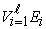from K and (z = a1) ∨ … ∨ (z = ), with size polynomial in s and ℓ.

In particular, if we can prove some contradiction from some collection K and xi = 0 as well as from K and xi = 1, then we can prove the contradiction from K and axiom xi = 0 ∨ xi = 1 of R(lin).

The use of this statement “allows to substitute” 0 or 1 instead of variable xi in collection K, but in order to prove contradiction from collection (1) we must do the substitution at least instead of 3 (m in common case) variables.

This statement is true for every n ≥ 1 and m from interval [1,2n-1], therefore if we denote by ¬φ’n the collections of ¬TTM’n,2n-1 (corresponding to collection (1) for φ’2), we have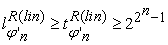So, both representations of hard-determinable tautologies φn as collections of disjuncts of linear equations require exponential proof complexities in R(lin).

### 4. Inference Rule to R(lin) System

Here we add some new inference rule to R(lin) and show that collections, constructed by analogy to (1) for ¬φ’n = ¬TTM’n,2n-1 have polynomially bounded proofs in supplemented system.

Renaming rule is given by figure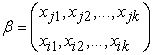and application of this rule to some disjuncts of linear equations consists in the replacing of variables xjs (1 ≤ s ≤ k) everywhere by the variables xjs (1 ≤ s ≤ k) (note that the renaming rule is not sound).

By R(lin)+renaming we denote the system R(lin), the set of inference rules of which is augmented by renaming rule.

For simplification of the proof of our main results we introduce some notations and prove some auxiliary propositions. Given n ≥ 1 and 1 ≤ j ≤ 2n-1 by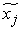we denote the sequence of variables x1j, x2j, …, xnj and for the following renaming rule we introduce the notations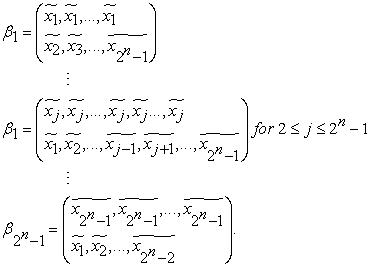Given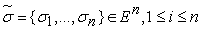and 1 ≤ j ≤ 2n-1 = 0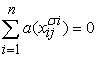from¬φ’n = ¬TTM’n,2n-1, can be presented as: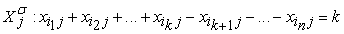, where k (0 ≤ k ≤ n) is the number of “1” in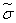.

Let for every π (1 ≤ π ≤ 2n-1)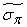be the binary n-component presentation of integer π, then the unsatisfiable collection for is the system of the following disjuncts of linear equations: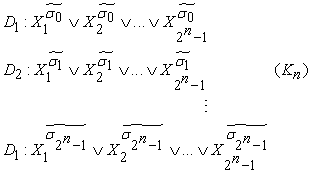4.1. Theorem 1

There exists polynomial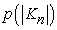such that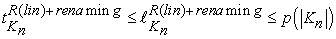.

Proof: The first -1 steps for the refuting of are the following: the applications of renaming rules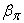to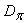(1 ≤ π ≤ 2n-1) give us the collection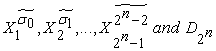(2)

The next steps are valid in R(lin).

Now let us prove 3 Lemmas.

Lemma 1: If some disjunct of linear equations A is refutable in R(lin) with the size at most s, then arbitrary disjunct of linear equation B is proved in R(lin) from A ∨ B with the size polynomial in s and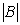.

Really, repeating all steps of some contradiction (0 = k) refutation from A to A ∨ B, we obtain (0 = k) ∨ B, and after using simplification rule we prove B.

Lemma 2: Given ℓ ≥ 1 and c ≥ 1 the equation A: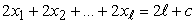has refutation in R(lin) with size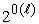.

From xi = 0 (xi = 1) 1 ≤ i ≤ ℓ using “+resolution”, we obtain 2xi = 0 (2xi = 2).

Using “-resolution” to A and 2x1 = 0 (2x1 = 2), we obtain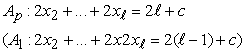By mentioned Lemma 4 from  from A and x1 = 0 ∨ x1 = 1 we prove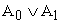Similarly from A0 ∨ A1 and x2 = 0 ∨ x2 = 1 we obtain A00 ∨ A10 ∨ A01 ∨ A11, where A00 (A10) –is “-resolution” result from A0 (A1) and 2x2 = 0, A01 (A11) – is “-resolution” result from A0 (A1) and 2x2 = 2.

Doing similar steps for all other variables, we prove Lemma 2.

Lemma 3: Given n ≥ 1 and 0 ≤ k ≤ n the collection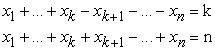has R(lin) refutation with size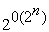.

Proof follows from Lemma 2 after using “+resolution” to both equations of given collection.

In order to finish the proof of Theorem we must use “+resolution” to every equation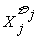and j-th equation from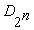, and then the Lemmas 1 – 3. Taking into consideration, that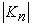is O(n 2n(2n - 1)), we prove the theorem.

Corollary: The system R(lin) +renaming has exponential speed-up over the system R(lin).

### 5. Conclusions

In this paper, we show that the strong proof-system R(lin), in which many of the outstanding examples of propositional tautologies have polynomially bounded proofs, is not super system; there exists a sequence of tautologies, which require proof complexity exponential in size of tautologies. We explored a new proof system R(lin)+renaming; which is stronger than R(lin): mentioned sequence of tautologies has polynomially bounded proof in this system. The introduced proof system R(lin)+renaming enables the polynomially upper bound for the second collections of disjuncts of linear equations. In addition to this, properties of R(lin) have been described that many of the “hard” provable in R outstanding examples of propositional tautologies (contradictions) have polynomially bounded proofs in R(lin). Finally, by way of polynomial simulation, an interesting theorem along with three lemmas in subsection 4.1 has been explored to focus the existence of a polynomialwith its special characteristics.

### References

  Aleksanyan S. R., Chubaryan A. A., “The polynomial bounds of proof complexity in Frege systems”, Siberian Mathematical Journal, Vol. 50, No. 2, 2009, pp. 243-249.In article CrossRef  Buss S.R., “Some remarks on lengths of propositional proofs”, Archive for Mathematical Logic, 34, 1995, pp. 377-394.In article CrossRef  Chubaryn An., “Relative efficiency of proof systems in classical propositional logic”, IZV. NAN Armenii, Mathematika, 37, No. 5, 2002, pp. 71-84.In article  Chubaryn An., Chubaryn Arm., Nalbandyan H., Sayadyan S., “A hierarchy of resolution systems with restricted substitution rules”, Computer Technology and Application, David Publishing, USA, vol. 3, No. 4, 2012, pp. 330-336.In article  Cook S.A., Reckhow A.R., “The relative efficiency of propositional proof systems”, Journal of Symbolic Logic, vol. 44, 1979, pp. 36-50.In article CrossRef  Johnsonbaugh R., Discrete Mathematics, Fifth Edition, Pearson Education Singapore, 2002.In article  Krajicek J., “On the weak pigeonhole principle”, Fundamentals of Mathematics, 170, 2001, pp. 123-140.In article CrossRef  Pudlak P. “Lengths of proofs” Handbook of proof theory, North-Holland, 1998, pp. 547-637.In article CrossRef  Raz Ran, Tzameret Iddo, “Resolution over linear equations and multilinear proofs”, Ann. Pure Appl. Logic 155 (3), 2008, pp. 194-224.In article CrossRef  Tseitin G. S. “On the complexity of derivation in the propositional calculus”, (in Russian), Zap. Nauchn. Semin. LOMI, Leningrad: Nauka, Vol. 8, 1968, pp. 234-259.In article